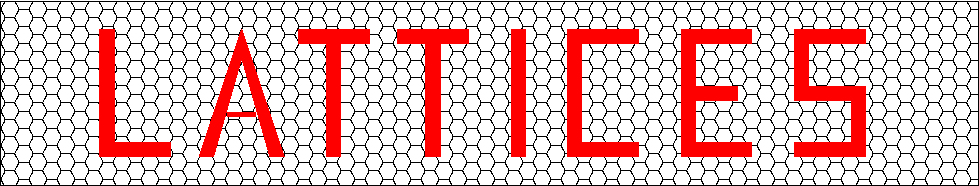## Catalogue of Lattices

This data-base of lattices is a joint project of
Neil J. A. Sloane (njasloane@gmail.com) and
Gabriele Nebe, RWTH Aachen University (nebe@math.rwth-aachen.de)

Our aim is to give information about all the interesting lattices in "low" dimensions.
Contributions are welcome.

For a list of the lattices in the data-base, see the Index file.

The files in the data-base are in two different formats:
(i) the standard format (with a file name such as E8.std), which is what we use internally; these files are in plain ASCII, which is the most convenient for computations; and
(ii) the html format (with a file name such as E8.html), which looks better on the World Wide Web; this is the format you will see when you download files from the data-base.

Several shell scripts are available for converting from the html format to other formats:

Each lattice is specified by some subset of the following properties

• %NAME (required)
• %DIMENSION (required)
• %DET (determinant)
• %TYPE
• %BASIS (basis for lattice; may have more than n coordinates)
• %TRIANGULAR_BASIS (triangular & square matrix giving basis for lattice)
• %GRAM (floating point or integer Gram matrix)
• %GRAM(MAPLE) (Gram matrix in Maple format)
• %GRAM(PARI) (Gram matrix in PARI format) (etc)
• %EIGENVALUES
• %DIVISORS (elementary divisors)
• %MINIMAL_NORM
• %DENSITY (center density)
• %HERMITE_NUMBER
• %KISSING_NUMBER
• %MINVECS
• %THETA_SERIES
• %COVERING_NORM (square of covering radius)
• %GLUE_VECTORS (representatives for L*/L )
• %GENUS
• %GROUP_ORDER
• %GROUP_NAME
• %GROUP_GENERATORS
• %HERMITIAN_STRUCTURE
• %HERMITIAN_GROUP_ORDER
• %HERMITIAN_GROUP_GENERATORS
• %PROPERTIES
INTEGRAL = 1 (lattices are real unless stated otherwise)
• %BACHER_POLYNOMIAL
• %REFERENCES
• %NOTES
• %URL (links to other sites for this lattice)
• %LAST_LINE (required)

For an explanation of (most of) these terms, see SPLAG:

"SPLAG" is an abbreviation for the book Sphere Packings, Lattices and Groups,
by J. H. Conway and N. J. A. Sloane, Springer-Verlag, NY, 1988; 3rd edition in preparation, 1996;

Convention: 0 = FALSE, 1 = TRUE

## Example: The E8 lattice

```%NAME	 	(obligatory)
E8
%DIM   		(obligatory)
8
%BASIS 	 	(optional)
8 8 		(no of rows, no of columns)
then 64 numbers giving entries in generator matrix
%TRIANGULAR_BASIS	(optional)
8 8		(no of rows, no of columns)
then 64 numbers giving entries in triangular and square generator matrix
%GRAM 		(optional)
8 8
then 64 numbers giving entries of Gram matrix

or

%GRAM		(optional)
8 0
then 8*9/2 = 36 numbers giving entries of Gram matrix in triangular format

%DET  		(optional)
1
%GLUE_VECTORS  		(optional)
nogl
then nogl numbers for the glue vectors (including the 0 glue word)
%PROPERTIES 		(optional)
INTEGRAL=1 		(means this is an integral lattice)
%MINIMAL_NORM		(optional)
2		(minimal norm)
%KISSING_NUMBER		(optional)
240 		(kissing number, i.e. number of minimal vectors)
%DENSITY		(optional)
.0625		(center density delta, Ref. SPLAG p. 13)
%HERM		(optional)
2		(Hermite number = 4 * delta^(2/dim) )
%NOTES 		(optional)
comment lines go here
%REFERENCES
SPLAG p. 120.
%LAST_LINE 		(obligatory last line)
```